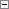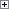Use this data type to contain a date formula that has the same capabilities as an ordinary input string for the CALCDATE Function (Date). The DateFormula data type is used to provide multilanguage capabilities to the CALCDATE Function (Date).Remarks

When a date calculation formula is stored in a DateFormula field, it is converted to a generic, non-language dependent format. When a date calculation formula is retrieved from a DateFormula field, it is converted to a valid date conversion string for the currently selected language.

To assign a value to a DateFormula data type, whether it is a field or a variable, you must use the EVALUATE Function.

Example

This example requires that you create a DateFormulaVariable variable that is a DateFormula data type.Copy Code
IF FORMAT(DateFormulaVariable) = ' ' THEN
EVALUATE(DateFormulaVariable, '1W');

You must use the FORMAT Function (Code, Text) to make a comparison with a text string. If you do not use this function, then the IF statement will fail because you cannot compare a DateFormula data type with a Text data type.